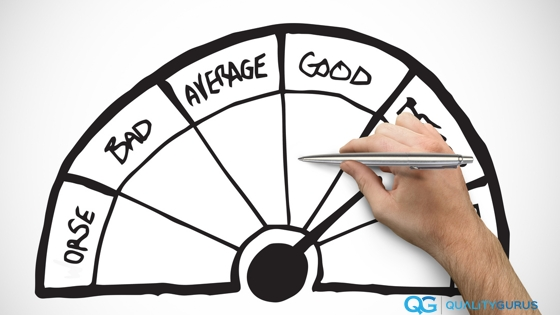# Top Three Measures of Central Tendency

Three commonly used measures of central tendency are: Mean, Median and Mode.

1. Mean:

Mean is also commonly known as the average, e.g., the average income of the country, the average height of people, etc.
The mean is the most commonly used measure of central tendency.

Formula: $$\bar{x} = \frac{1}{n}\left (\sum_{i=1}^n{x_i}\right ) = \frac{x_1+x_2+\cdots +x_n}{n}$$

Example: Find the mean of 10, 11, 14, 9 and 6.

Add all these numbers and divide that by the number of items.

$$Mean = \frac{(10+11+ 14+ 9 +6)}{5} = \frac{50}{5} = 10$$

Mean of the population is represented by $$\mu$$ and the Mean of a sample is represented by $$\bar{x}$$.

The problem with the Mean is that it is affected by extreme values and that makes other methods (Mode and Median), sometimes more important than Mean.

For example, if in the above example, instead of 14 if you have 140, this is going to change the value of Mean drastically.

2. Median:

Unlike Mean, the Median is not affected by the extreme value into the data.

To calculate the Median, you need to put your data in ascending or descending order. The Median is the middle number in the arranged data.

Example 1: Find the median of 10, 11, 14, 9 and 6.

Step 1: Arrange the data in ascending order (or descending order, if you want).

That gives us 6, 9, 10, 11 and 14

Step 2: In this sequence 10 is the middle number; hence the Median is 10.

The above was the case when your data had the odd number of readings. Let’s look at another example where you have even number of readings.

Example 2: Find the median of 10, 11, 14, 9, 6, 11.

Step 1: Arrange the data in ascending order (or descending order, if you want).

It gives us 6, 9, 10, 11, 11, 14.

Step 2: In this sequence, there is no single number in the middle. Hence we find the average of two numbers (10 and 11) in the middle number; hence the Median is 10.5

And as I earlier said that the Median is not affected by extreme values. For example in this case also instead of 14, if it were 140, that wouldn’t have made any difference to the value of the Median.

3. Mode:

The Mode is the most frequently occurring value.

Example: Find the mode of 10, 11, 14, 9, 6 and 10

The number 10 is occurring the most of times in the above data hence 10 is the mode.

#### Six Sigma Black Belt

• Course based on ASQ CSSBB Body of Knowledge.

• 30+ hours of videos, quizzes and notes

• Get this $200 course for just$49.99 today.